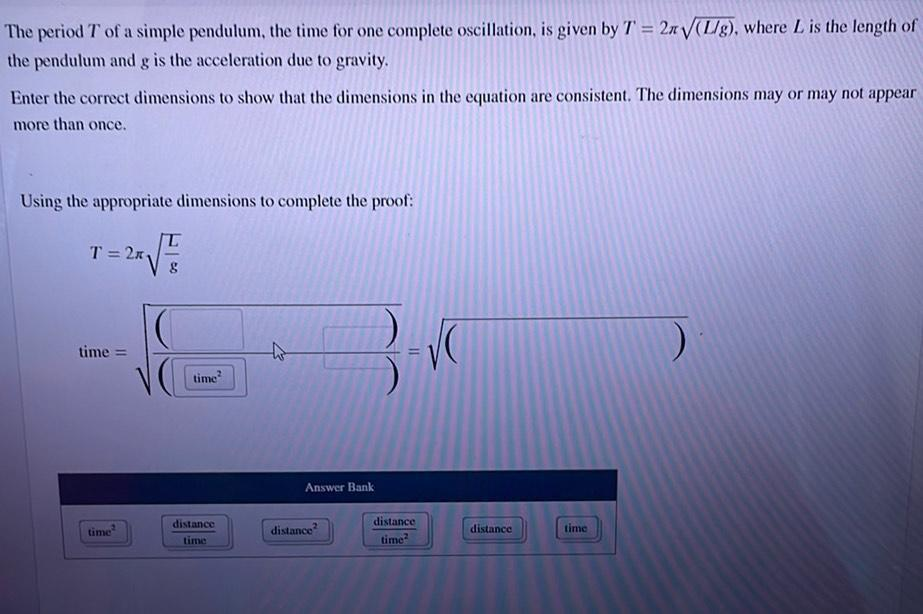Question:

# The period T of a simple pendulum, the time for one complete oscillation, is given by T = 27(L/g), where L is the length of theThe period T of a simple pendulum, the time for one complete oscillation, is given by T = 27(L/g), where L is the length of the pendulum and g is the acceleration due to gravity. Enter the correct dimensions to show that the dimensions in the equation are consistent. The dimensions may or may not appear more than once. Using the appropriate dimensions to complete the proof: T T = 2x 8 time = 3 VO time? Answer Bank time? distance time distance? distance time? distance time